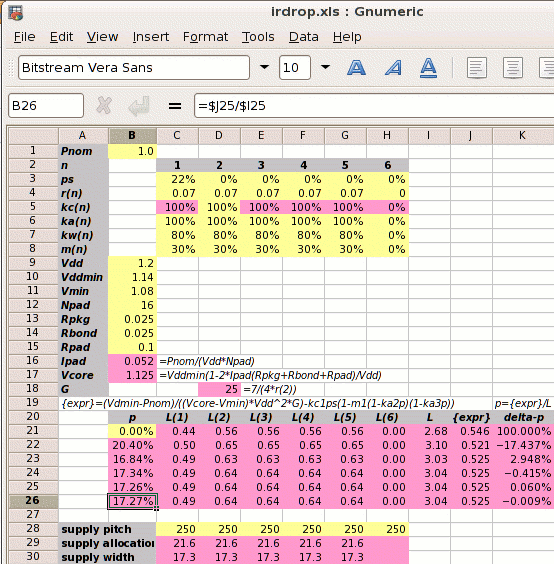This example is the previous one with 30% of the core area blocked to all metal routing because of fixed blocks. This 30% is the core area before adding power straps.

Step 1: Calculate Ipad and Vcore:

1⁄(1.2×16) = 0.052A
Vcore =
1.14×(1−2×0.052×(0.025+0.025+0.1)⁄1.2
1.125V

Step 2: Calculate the reference power supply conductance G:

G =
 7 4×r2

7 ⁄ (4 × 0.07) =  25 mhos

Step 3 is to set out the values of kan, kwn, kcn and mn for each metal layer, and use these to calculate the value of L.

metal layer 1 2 3 4 5
kan 100% 100% 100% 100% 100%
power metal allocated coefficient
kwn  80%  80%  80%  80%  80%
power metal used coefficient
kcn 100% 100% 100% 100% 100%
conductivity coefficient
mn  30%  30%  30%  30%  30%
core area blocked
kan and kcn are 100% because all power straps have the same space allocated and the metal resistivities are the same. kwn is 80% for all metal layers because the power strap widths and spacings are also all the same.

The value of L depends on p which we don't know. We iterate to the solution and use p=0 for the first estimate.

 L = kw1kc1(1-ps)(1-m1(1-ka2p)(1-ka3p))+ kw2kc2(1-m2(1-ka2p)(1-ka3p))+ kw3kc3(1-m3(1-ka2p)(1-ka3p))+ kw4kc4(1-m4(1-ka2p)(1-ka3p))+ kw5kc5(1-m5(1-ka2p)(1-ka3p)) = ( 0.44 + 0.56 + 0.56 + 0.56 + 0.56 ) = 2.68

Step 4: Calculate the power strap allocation percentage p. The solution must be iterated, and the calculation below shows the first iteration.

 m1′ = m1×(1-ka2p)(1-ka3p)
p =
 { Vddmin×Pnom −kc1×ps(1-m1′) } × 1 (Vcore−Vmin)×Vdd2×G L
 { 1.14×1 −1×0.22×(1-0.3) } × 1 (1.125−1.08)×1.22×25 2.68
(0.701−0.156)×0.374 = 20.40%

As shown on the right, a spreadsheet can be used to iterate to the answer of p=17.27%.

The presence of the fixed blocks has increased the percentage of metal which must be allocated to power straps from 12.53% to 17.27%, an increase of 38%.

Step 5: Calculate the new core size. If the initial core size estimate without power straps is x, then with power straps the core size becomes x

 x′ = x = x = x = x+20.88% √(((1-ka2p)(1-ka3p)) √0.82732 0.8273

The value 20.88% is called the IR Drop Adder.

Let us look at a more complex example.

Design Attribute Value
Pnom core power consumption 1W
ps fraction of metal-1 in the standard cells used for power supplies 22% (for vsclib)
rn resistivity of metal layer n in ohms per square 0.07Ω per sq.
kan
 user defined   ratio of metal layer n allocated to power metal-2 allocated to power
100%
kwn
 user defined   ratio of metal layer n used for power metal-2 allocated to power
80%
mn percentage of metal layer n blocked to power straps 30%
Vdd the nominal supply voltage 1.2V
Vddmin the minimum supply voltage, 5% less than nominal 1.14V
Vmin the desired voltage at the centre of the die, 10% less than the nominal 1.08V
Rpkg the resistance of the package leadframe 25mΩ
Rbond the resistance of the bond wire 25mΩ